# Racing track

On the racing track circling three cars. The first pass one circuit for 8 seconds, the second for 20 seconds, and a third for 8 seconds.

a) Calculate the number of seconds since starting to catch all three cars together for the first time again across the start line?

b) How many laps ran each car until catch all three cars together for the first time?

a =  40 s
1.:  5
2.:  2
3.:  5

### Step-by-step explanation:

$1.....40\mathrm{/}8=5$
$2.....40\mathrm{/}20=2$
$3.....40\mathrm{/}8=5$Did you find an error or inaccuracy? Feel free to write us. Thank you!Tips to related online calculators
Do you want to calculate least common multiple two or more numbers?
Do you want to convert time units like minutes to seconds?

## Related math problems and questions:

• Two cars on ring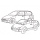There were two cars on the round track (ring) in the adjacent tracks, the first car in the inner track, the second in the outer track. Both cars started at the same time from one starting track. The first toy car drove every four laps simultaneously as th
• Runners circle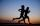Pepa circles the track in 36 seconds. Kamil in 42 seconds. They started together. How many seconds will meet again at the start?
• A number 5A number is divisible by 24, 25, and 120 if it is increased by 20. Find the number.
• LCMWhat is the least common multiple of 5, 50, 14?
• The Nürburgring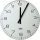The Nürburgring in Germany is a racetrack that hosts racing events all year. Two of the fastest laps ever driven on the track are 6 minutes 47.30 seconds and 6 minutes 52.01 seconds. How much faster is the 6 minutes 47.30 seconds time than the 6 minutes 5
• Lcm = 22 + gcdThe least common multiple of two numbers is 22 more than their greatest common divisor. Find these numbers.
• Ships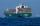Red ship begins its circuit every 30 minutes. Blue boat begins its circuit every 45 minutes. Both ships begin their sightseeing circuit in the same place at the same time always at 10:00 o'clock. a / What time does meet boat again? b / How many times a da
• Three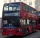Three buses follow the same circular route. The first driver is the slowest because he has many stops, and it takes him 90 minutes to cross the route. The second driver will pass the circuit in 1 hour. The third driver has the fewest stops, and the circui
• Gcd and lcm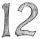Calculate the greatest common divisor and the least common multiple of numbers. a) 16 and 18 b) 24 and 22 c) 45 and 60 d) 36 and 30
• Per shiftTwo cars take beets from the same field to the sugar factory. Both drive at the same average speed. A car with mechanical tipping needs 15 minutes to travel to the sugar factory and back. A car that does not have a mechanical tipping needs 6 minutes longe
• Lcm to eliminate yIdentify the least common multiple that would eliminate the y-variable. 6x - 5y = -4 4x + 2y = 28
• Bus linesBuses connections are started from the bus stop on its regular circuit: No. 27 bus every 27 minutes and No.18 every half hour. What time started these two bus lines run if the bus stop met at 10:15 am.?
• Find unknown numberWhat is the number between 50 and 55 that is divisible by 2,3,6,9?
• Two gears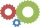Two gears with 13 and 7 teeth rotate locked into each other. How many turns does a big wheel have to make for both wheels to be in the starting position again?
• Lcm of three numbersWhat is the Lcm of 120 15 and 5
• Apples and pearsMom divided 24 apples and 15 pears to children. Each child received the same number of apples and pears - same number as his siblings. How many apples (j=?) and pears (h=?) received each child?
• A ropeA rope can be cut into equal length with no rope left over. The lengths can be 15cm,18cm or 25cm. What is the shortest possible length of the rope?